### Active Set Methods

The parameter vector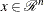may be subject to a set oflinear equality and inequality constraints: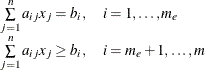The coefficients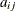and right-hand sides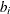of the equality and inequality constraints are collected in thematrix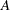and thevector.

Thelinear constraints define a feasible region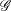in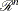that must contain the point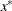that minimizes the problem. If the feasible regionis empty, no solution to the optimization problem exists.

All optimization techniques in PROC NLP (except those processing nonlinear constraints) are active set methods. The iteration starts with a feasible point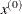, which either is provided by the user or can be computed by the Schittkowski and Stoer (1979) algorithm implemented in PROC NLP. The algorithm then moves from one feasible point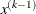to a better feasible point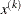along a feasible search direction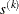: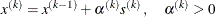Theoretically, the path of pointsnever leaves the feasible regionof the optimization problem, but it can hit its boundaries. The active set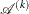of pointis defined as the index set of all linear equality constraints and those inequality constraints that are satisfied at. If no constraint is active for, the point is located in the interior of, and the active set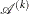is empty. If the pointin iterationhits the boundary of inequality constraint, this constraintbecomes active and is added to. Each equality or active inequality constraint reduces the dimension (degrees of freedom) of the optimization problem.

In practice, the active constraints can be satisfied only with finite precision. The LCEPSILON=option specifies the range for active and violated linear constraints. If the pointsatisfies the condition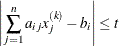where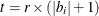, the constraintis recognized as an active constraint. Otherwise, the constraintis either an inactive inequality or a violated inequality or equality constraint. Due to rounding errors in computing the projected search direction, error can be accumulated so that an iteratesteps out of the feasible region. In those cases, PROC NLP may try to pull the iterateinto the feasible region. However, in some cases the algorithm needs to increase the feasible region by increasing the LCEPSILON=value. If this happens it is indicated by a message displayed in the log output.

If you cannot expect an improvement in the value of the objective function by moving from an active constraint back into the interior of the feasible region, you use this inequality constraint as an equality constraint in the next iteration. That means the active set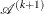still contains the constraint. Otherwise you release the active inequality constraint and increase the dimension of the optimization problem in the next iteration.

A serious numerical problem can arise when some of the active constraints become (nearly) linearly dependent. Linearly dependent equality constraints are removed before entering the optimization. You can use the LCSINGULAR= option to specify a criterionused in the update of the QR decomposition that decides whether an active constraint is linearly dependent relative to a set of other active constraints.

If the final parameter setis subjected to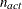linear equality or active inequality constraints, the QR decomposition of the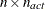matrix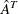of the linear constraints is computed by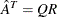, where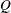is anorthogonal matrix and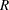is anupper triangular matrix. Thecolumns of matrixcan be separated into two matrices,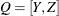, where
contains the firstorthogonal columns ofand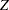contains the last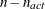orthogonal columns of. The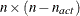column-orthogonal matrixis also called the nullspace matrix of the active linear constraints. The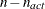columns of thematrixform a basis orthogonal to the rows of the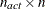matrix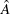.

At the end of the iteration process, the PROC NLP can display the projected gradient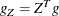In the case of boundary constrained optimization, the elements of the projected gradient correspond to the gradient elements of the free parameters. A necessary condition forto be a local minimum of the optimization problem is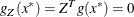The symmetric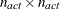matrix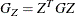is called a projected Hessian matrix. A second-order necessary condition forto be a local minimizer requires that the projected Hessian matrix is positive semidefinite. If available, the projected gradient and projected Hessian matrix can be displayed and written in an OUTEST= data set.

Those elements of thevector of first-order estimates of Lagrange multipliers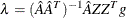which correspond to active inequality constraints indicate whether an improvement of the objective function can be obtained by releasing this active constraint. For minimization (maximization), a significant negative (positive) Lagrange multiplier indicates that a possible reduction (increase) of the objective function can be obtained by releasing this active linear constraint. The LCDEACT=option can be used to specify a thresholdfor the Lagrange multiplier that decides whether an active inequality constraint remains active or can be deactivated. The Lagrange multipliers are displayed (and written in an OUTEST= data set) only if linear constraints are active at the solution. (In the case of boundary-constrained optimization, the Lagrange multipliers for active lower (upper) constraints are the negative (positive) gradient elements corresponding to the active parameters.)